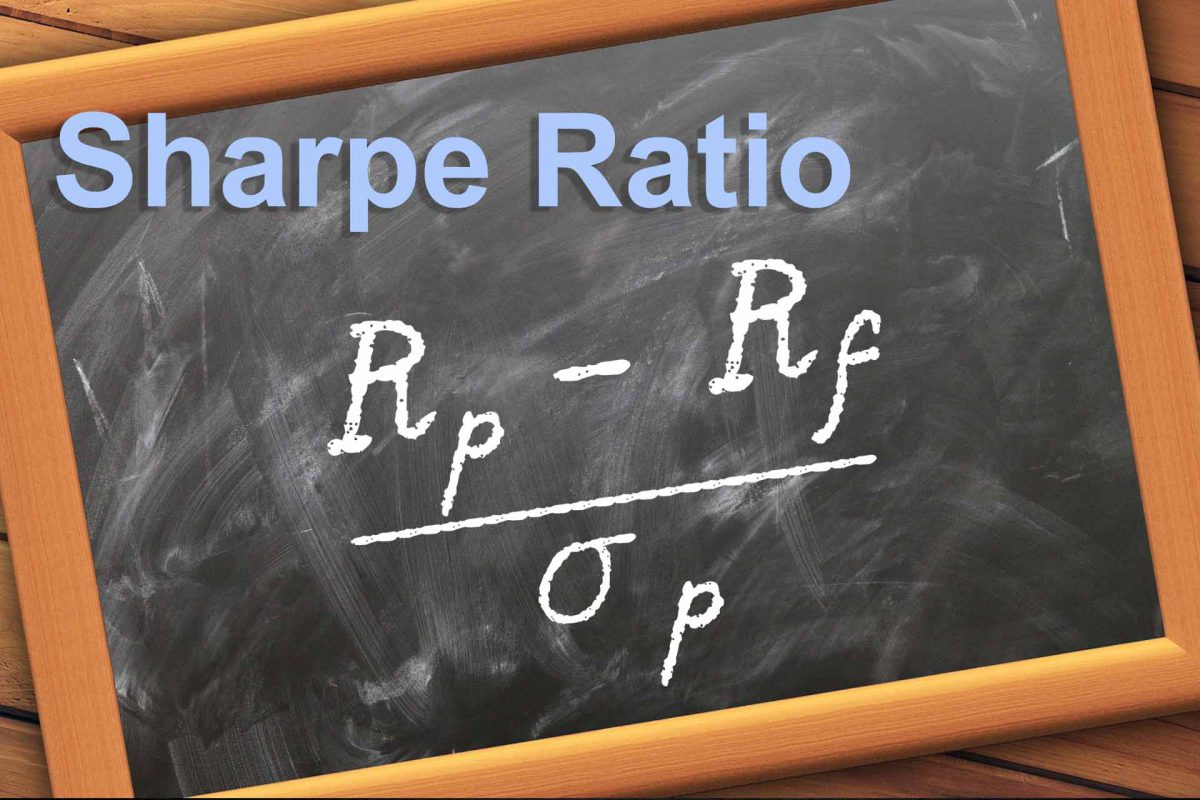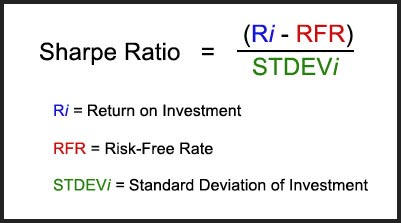What is Sharpe Ratio?Performance Analysis

When analyzing a given investment’s performance – be it an individual stock or a broad market index – many investors focus on the return produced over a certain time period. For instance, an investor might judge how well Apple common stock performed by looking at the total return over the last calendar year. This is referred to as a holding-period return. Hypothetically, let us say that Apple was up 10% over the last year. A solid return, no doubt. However, this level of analysis considers absolute return only, without regard for the corresponding level of risk associated with the investment. It is a surface-level analysis.

While the 10% return described above is attractive, astute investors should take their analysis one step further by incorporating risk into the framework. Risk and return are joined at the hip: investors cannot achieve outsized returns without assuming outsized risk. All else equal, investors would prefer to get as much return as possible for as little risk as possible. Thus, when presented with a menu of investments that could potentially earn 10%, the investor would choose the investment with the lowest risk. Although it can take many forms, risk is most commonly measured by the standard deviation metric. Statistically, the standard deviation of returns is the variability or volatility of returns around the average. The higher the standard deviation, the riskier the investment.

Calculating the Sharpe Ratio

Since investors will always choose the highest returning investment for a given level of risk, they should incorporate reward-to-risk ratios into performance analysis to get a clearer picture of their experience in the investment. There are several such ratios, but the most commonly used metric is called the Sharpe Ratio. The metric was conceived by the economist William Sharpe, for which he won a Nobel Prize. The numerator begins with the return on a given investment and subtracts the risk-free rate (RFR) of return. In most cases, the RFR is assumed to be either cash or short-term US government treasury bonds as they are seen as having almost no possibility of default (hence being risk-free). This is also referred to as the excess return as it displays any return over and above the RFR.

The numerator is then divided by the investment’s standard deviation, and the mathematical result is the investment’s Sharpe Ratio for a given holding period. Visually, the Sharpe Ratio can be displayed as:Benefits and Limitations of the Sharpe Ratio

The ratio should be interpreted as a risk-adjusted measure of performance, whereby an investor can standardize a variety of investments by risk and make an apples-to-apples comparison. The ratio can also be thought of as a measure of statistical efficiency, displaying to the investor how much return is produced for a given level of risk. All else equal, the higher the Sharpe Ratio, the better.

The beauty of the Sharpe Ratio lies in its simplicity. With just one simple quotient, the Sharpe Ratio can cut through the noise and communicate to investors how much bang they are getting for each buck risked. Higher Sharpe Ratio investments are more efficient, statistically speaking, and should therefore be preferred to lower Sharpe Ratio investments.

However, investors should be weary of relying too heavily on the Sharpe Ratio alone when analyzing investment risk. There are several limitations to the Sharpe Ratio. First, risk may not be the prevailing factor that matters for a given investment. Volatility, as measured by standard deviation, may take a back seat to absolute return or other measures of investment performance such as CAGR (compound annual growth rate). In this case, a lower-returning investment may have a high Sharpe Ratio as a result of lower risk, but that lower volatility comes associated with lower overall returns. For investors that are willing and able to stomach some degree of risk, choosing investments with high Sharpe Ratios may result in foregone future returns.

Second, risk and volatility are not solely downside measures. Volatility works on both the upside and the down, and an investment with a high Sharpe Ratio (low volatility) may give up considerable upside for a smoother, less volatile ride. It depends on the investor’s definition of risk and how much they are willing to accept. It may be more appropriate for an investor to consider measures of downside risk only, such as upside / downside capture or the Sortino Ratio, when comparing investment choices.

The data used to calculate Sharpe Ratios are always backward looking and thus do not provide any insight into expected future returns. It is important to note that past performance is not necessarily indicative of future results, and no investor can say for sure what will happen in the future.

Finally, the ratio is less meaningful when analyzing individual investments and trades. Instead, it is optimal to compare Sharpe Ratios within a larger portfolio of numerous, diverse investments. Indeed, the originator of the ratio, William Sharpe himself, has claimed that the metric ought to be used primarily for an entire portfolio, as in some cases an investment with a low Sharpe Ratio may be more beneficial to the portfolio in entirety. After all, what really matters is how each investment interacts with each other within a larger, complete portfolio, and that is where the power of the Sharpe Ratio comes into play.

Nevertheless, the Sharpe Ratio is an excellent descriptor of the interaction of return and risk for a given investment over a given time period. It is a great starting point to consider the range of expected future risk and return scenarios. When applied to complete portfolios, it is likely that Sharpe Ratio considerations could result in better decisions about the tradeoff between return and risk. However, investors should avoid using the Sharpe Ratio in isolation, and should avoid falling into the trap of using a backward-looking statistical measure such as the Sharpe Ratio to precisely forecast the future.